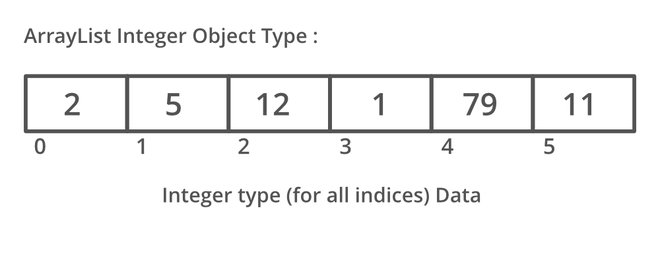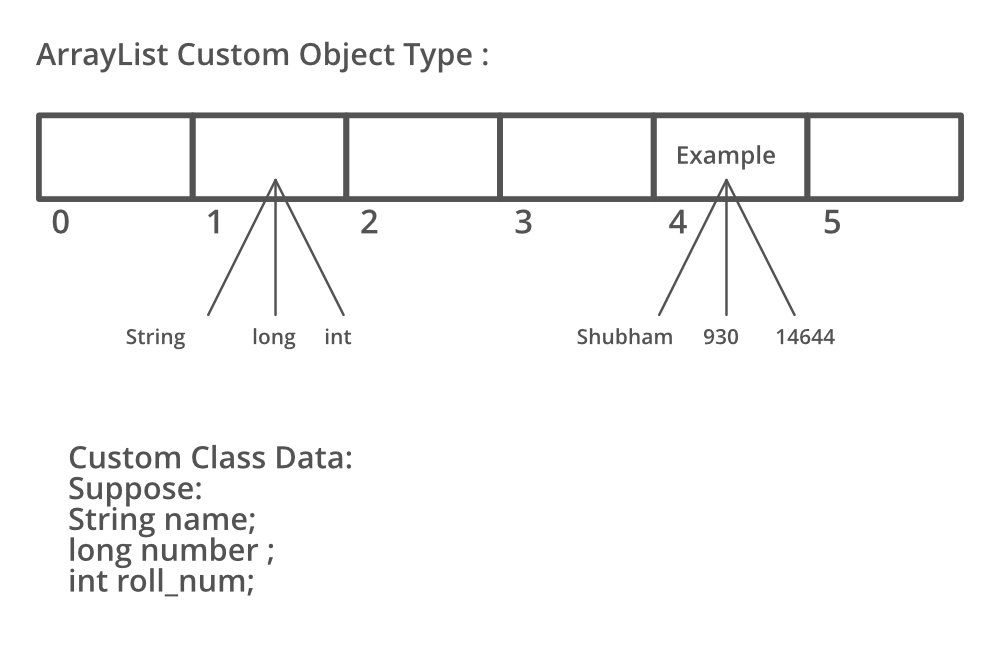GeeksforGeeks App
Open AppBrowser
Continue

# Custom ArrayList in Java

Before proceeding further let us quickly revise the concept of the arrays and ArrayList quickly. So in java, we have seen arrays are linear data structures providing functionality to add elements in a continuous manner in memory address space whereas ArrayList is a class belonging to the Collection framework. Being a good programmer one is already aware of using ArrayList over arrays despite knowing the differences between these two. Now moving ahead even with ArrayList there comes a functionality to pass the type of datatype of elements that are supposed to be stored in the ArrayList be it an object, string, integer, double, float, etc.

Syntax:

`Arraylist<DataType> al = new ArrayList<Datatype> ;`

Note: ArrayList in Java (equivalent to vector in C++) having dynamic size. It can be shrunk or expanded based on size. ArrayList is a part of the collection framework and is present in java.util package

Syntax:

`ArrayList <E> list = new ArrayList <> ();`

The important thing here out is that E here represents an object datatype imagine be it Integer here. The Integer class wraps a value of the primitive type int in an object. An object of type Integer contains a single field whose type is int. Do go through the concept of wrapper classes in java before moving forward as it will serve here at the backend making understanding clearer if we are well aware of autoboxing and unboxing concepts. It is because while performing operations over elements in list their syntax will differ so do grasping over concept will deplete as suppose consider a scenario of adding elements to custom ArrayList and note the differences in syntax between two of them.

Syntax:

```ArrayList<Integer> al = new Arraylist<Integer>() ;

Syntax:

```ArrayList alobj = new Arraylist() ;
alobj(new Integer(1)) ;```

Let us take a sample illustration to perceive as provided below as follows:

Illustration:here we are having all the elements of the same type which in general we often do use. Now let us propose the same diagrammatic flow ArrayList simply supports multiple data in the way shown in this image.In the above ArrayList, we can clearly see that the elements been stored in are of different types. So it does erupt out the concept of restricting. to a single type and not only this List do go gives us the flexibility to make List as per our type where we have access to what kind of data types can be there in our ArrayList. This List is referred to as Custom ArrayList in java. A custom ArrayList has attributes based on user requirements and can have more than one type of data. This data is provided by a custom inner class which is formed by the combination of various primitive object datatypes.

Implementation: Consider a case when we have to take input as N number of students and details are:

• roll number
• name
• marks
• phone number

Suppose if we are unaware of the concept of custom Arraylist in java then we would be making below listed individual ArrayLists. As we define 4 ArrayLists and save data accordingly in each of them.

`ArrayList<Integer> roll = new ArrayList<>();  // roll number`
` ArrayList<String> name = new ArrayList<>(); // name`
`ArrayList<Integer> marks = new ArrayList<>(); //  marks`
`ArrayList<Long> phone = new ArrayList<>();  // phone number `

Now we would be iterating over each of them to fetch student data increasing the time complexity of our program to a greater extent as illustrated below as follows.

```for (int i = 0; i < n; i++)
{

// Adding all the values to each arraylist
// each arraylist has primitive datatypes

}```

Now let us do the same with the help of the concept learned above by implementing the same. So in order to construct our custom ArrayList perform the below-listed steps as follows:

Procedure: Constructing custom ArrayList are as follows:

1. Build an ArrayList Object and place its type as a Class Data.
2. Define a class and put the required entities in the constructor.
3. Link those entities to global variables.
4. Data received from the ArrayList is of that class type that stores multiple data.

Example

## Java

 `// Java program to illustrate Custom ArrayList` `// Importing ArrayList class from java.util package``import` `java.util.ArrayList;` `// Class 1``// Outer class``// Main class``// CustomArrayList``class` `Data {` `    ``// Global variables of the class``    ``int` `roll;``    ``String name;``    ``int` `marks;``    ``long` `phone;` `    ``// Constructor has type of data that is required``    ``Data(``int` `roll, String name, ``int` `marks, ``long` `phone)``    ``{` `        ``// Initialize the input variable from main``        ``// function to the global variable of the class` `        ``// this keyword refers to current instance``        ``this``.roll = roll;``        ``this``.name = name;``        ``this``.marks = marks;``        ``this``.phone = phone;``    ``}``}``public` `class` `GFG {` `    ``// Custom class which has data type class has``    ``// defined the type of data ArrayList``    ``// size of input 4``    ``int` `n = ``4``;` `    ``// Class 2``    ``// Inner class``    ``// The custom datatype class` `    ``public` `void` `addValues(``int` `roll[], String name[],``                          ``int` `marks[], ``long` `phone[])``    ``{``        ``// local custom arraylist of data type``        ``// Data having (int, String, int, long) type``        ``// from the class``        ``ArrayList list = ``new` `ArrayList<>();` `        ``for` `(``int` `i = ``0``; i < n; i++) {``            ``// create an object and send values to the``            ``// constructor to be saved in the Data class``            ``list.add(``new` `Data(roll[i], name[i], marks[i],``                              ``phone[i]));``        ``}` `        ``// after adding values printing the values to``        ``// test the custom arraylist``        ``printValues(list);``    ``}` `    ``// Method 2``    ``// To print the values``    ``public` `void` `printValues(ArrayList list)``    ``{` `        ``// list- the custom arraylist is sent from``        ``// previous function` `        ``for` `(``int` `i = ``0``; i < n; i++) {` `            ``// Data received from arraylist is of Data``            ``// type which is custom (int, String, int,``            ``// long) based on class Data``            ``Data data = list.get(i);` `            ``// Print and display custom ArrayList``            ``// elements that holds for student attribute` `            ``// Data variable of type Data has four``            ``// primitive datatypes roll -int name-``            ``// String marks- int phone- long``            ``System.out.println(data.roll + ``" "` `+ data.name``                               ``+ ``" "` `+ data.marks + ``" "``                               ``+ data.phone);``        ``}``    ``}``    ``// Method 1``    ``// Main driver method``    ``public` `static` `void` `main(String args[])``    ``{` `        ``// Custom input data``        ``int` `roll[] = { ``1``, ``2``, ``3``, ``4` `};``        ``String name[]``            ``= { ``"Shubham"``, ``"Atul"``, ``"Ayush"``, ``"Rupesh"` `};``        ``int` `marks[] = { ``100``, ``99``, ``93``, ``94` `};``        ``long` `phone[] = { 8762357381L, 8762357382L,``                         ``8762357383L, 8762357384L };` `        ``// Creating an object of the class``        ``GFG custom = ``new` `GFG();` `        ``// Now calling function to add the values to the``        ``// arraylist``        ``custom.addValues(roll, name, marks, phone);``    ``}``}`

Output

```1 Shubham 100 8762357381
2 Atul 99 8762357382
3 Ayush 93 8762357383
4 Rupesh 94 8762357384```

A custom ArrayList in Java can be created by extending the java.util.AbstractList class and implementing its methods. Here’s an example of how you can create a custom ArrayList:

## Java

 `import` `java.util.AbstractList;``import` `java.util.Arrays;``import` `java.util.List;` `public` `class` `CustomArrayList ``extends` `AbstractList {``    ``private` `int` `size = ``0``;``    ``private` `static` `final` `int` `DEFAULT_CAPACITY = ``10``;``    ``private` `Object elements[];` `    ``public` `CustomArrayList() {``        ``elements = ``new` `Object[DEFAULT_CAPACITY];``    ``}` `    ``public` `CustomArrayList(``int` `capacity) {``        ``elements = ``new` `Object[capacity];``    ``}` `    ``@Override``    ``public` `int` `size() {``        ``return` `size;``    ``}` `    ``@Override``    ``public` `E get(``int` `index) {``        ``if` `(index >= size || index < ``0``) {``            ``throw` `new` `IndexOutOfBoundsException(``"Index: "` `+ index + ``", Size "` `+ index);``        ``}``        ``return` `(E) elements[index];``    ``}` `    ``@Override``    ``public` `void` `add(``int` `index, E element) {``        ``if` `(index > size || index < ``0``) {``            ``throw` `new` `IndexOutOfBoundsException(``"Index: "` `+ index + ``", Size "` `+ index);``        ``}``        ``ensureCapacity();``        ``for` `(``int` `i = size - ``1``; i >= index; i--) {``            ``elements[i + ``1``] = elements[i];``        ``}``        ``elements[index] = element;``        ``size++;``    ``}` `    ``@Override``    ``public` `E remove(``int` `index) {``        ``if` `(index >= size || index < ``0``) {``            ``throw` `new` `IndexOutOfBoundsException(``"Index: "` `+ index + ``", Size "` `+ index);``        ``}``        ``Object item = elements[index];``        ``for` `(``int` `i = index; i < size - ``1``; i++) {``            ``elements[i] = elements[i + ``1``];``        ``}``        ``size--;``        ``return` `(E) item;``    ``}` `    ``private` `void` `ensureCapacity() {``        ``int` `newSize = elements.length * ``2``;``        ``elements = Arrays.copyOf(elements, newSize);``    ``}` `    ``public` `static` `void` `main(String[] args) {``        ``List list = ``new` `CustomArrayList<>();``        ``list.add(``1``);``        ``list.add(``2``);``        ``list.add(``3``);``        ``System.out.println(``"CustomArrayList: "` `+ list);``    ``}``}`

Output

`CustomArrayList: [1, 2, 3]`

In this example, the custom ArrayList is created by extending the AbstractList class and implementing its methods size, get, add, and remove. The custom ArrayList also has a private method called ensureCapacity which doubles the size of the ArrayList if it runs out of space.

### Advantages of using a custom ArrayList in Java:

1. Flexibility: Creating a custom ArrayList allows you to customize the behavior of the ArrayList to meet the specific needs of your application.
2. Understanding: Creating your own ArrayList from scratch can help you understand how ArrayLists work and how to use them effectively.

### Disadvantages of using a custom ArrayList in Java:

1. Time consumption: Creating a custom ArrayList can be time-consuming and requires a good